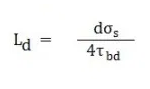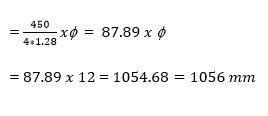# Development Length (Ld) - Quick Calculation of Development Length on Site, Anchorage Length, Formula of Development Length

Development Length (Ld)

Development Length is known as the minimum length of reinforcement bar needed to provide embedded into the concrete column to maintain and establish the required bond & design strength between concrete and reinforcement bar.Development Length

What is the use of development length, reason for providing development length ?
• To maintain a required and safest bond between the reinforcement bar surfaces which is embedded with column and the concrete, so that the failure due to slippage of reinforcement bar not occurs during maximum load.
• Development length (Ld) is extra length of the bar provided as development length which is responsible for transferring the stresses developed in section to the adjoining sections which is junction of beam and column
• Provision of appropriate development length (Ld) is an important aspect of safe construction practices.
• Proper development length in reinforcement bars shall be provided as per the steel grade (i.e. Fe 400, 450, 500, 550, 600) considered in design.
Formula for Development Length:Formula for LD
Where,
f    = Nominal Diameter of Reinforcement Bar
ss    = Stress in Bar at a section considered at design load
tbd  = Design Bond Stress

Calculation of Development Length:
There are two method of calculation of development bar length which are given below :
1     .      Limit State method
2     .      Working Stress method
Calculation of Development length for M20 grade concrete with Steel Fe 450 f N/mm2  from limit state method.
Now,
Dia of bar f = 12 mm
Stress in bar (ss) = 450 N/mm2 (Fe 450 steel)
Design Bond Stress (tbd ) = 1.28 N/mm2  (for M20 as per IS : 456-200 for deformed bars)calculation for Ld

Generally, in the field for quick practice, the development length requirement is calculated by ‘41 times Ø’ or ‘41 Ø
Where,
41 is the factor calculated using the above formula & Ø is the dia of the bar.

Anchorage Length:
Anchorage length is one type of development length also which is provided inside the fixed or support end. Which varies on  Dia or angle of bar which we have to bent, when the angle decrees anchorage length increase or vice versa.  It is 4 times dia of bar for 180 Degree bend,6 times for 135 degree and 8 times for 90 degree bend of bar.Anchorage Length

Tags :
Development Length (Ld)
Calculation of Development Length on Site,
Anchorage Length,
Formula of Development Length,
reason for Development Length,